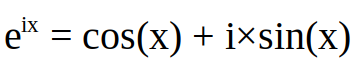# Math Contest #22 [2 SBI]Here you can keep your brain fit by solving math related problems and also earn SBI or sometimes other rewards by doing so.
The problems usually contain a mathematical equation that in my opinion is fun to solve or has an interesting solution.
I will also only choose problems that can be solved without additional tools(at least not if you can calculate basic stuff in your head), so don't grab your calculator, you won't need it.
↓↑↓↑↓↑↓↑↓↑↓↑↓↑↓↑↓↑↓↑↓↑↓↑↓↑↓↑↓↑↓↑↓↑↓↑↓↑↓↑↓↑↓↑↓↑↓↑↓

# Rules

#### You have 4 days to solve it.

↓↑↓↑↓↑↓↑↓↑↓↑↓↑↓↑↓↑↓↑↓↑↓↑↓↑↓↑↓↑↓↑↓↑↓↑↓↑↓↑↓↑↓↑↓↑↓↑↓

# Problem

Today I want to try something different: A proof.

Snice I don't know how much experience you have in mathematical proofs I will ignore small logical or syntactical errors in prize distribution.

Proof to me that euler's formula is true:Tip: You might want to take a look at taylor series.

↓↑↓↑↓↑↓↑↓↑↓↑↓↑↓↑↓↑↓↑↓↑↓↑↓↑↓↑↓↑↓↑↓↑↓↑↓↑↓↑↓↑↓↑↓↑↓↑↓

To everyone who already participated in a past contest, come back today and try a new problem(tell me if you don't want to be tagged):
@addax @ajayyy @athunderstruck @bwar @contrabourdon @crokkon @fullcoverbetting @golddeck @heraclio @hokkaido @iampolite @kaeserotor @masoom @mmunited @mobi72 @mytechtrail @ninahaskin @onecent @rxhector @sidekickmatt @sparkesy43 @syalla @tonimontana @vote-transfer @zuerich

In case no one gets a result(which I doubt), I will give away the prize to anyone who comments.

↓↑↓↑↓↑↓↑↓↑↓↑↓↑↓↑↓↑↓↑↓↑↓↑↓↑↓↑↓↑↓↑↓↑↓↑↓↑↓↑↓↑↓↑↓↑↓↑↓

@contrabourdon sponsors my contests with 2 STEEM weekly.
You can support him by using a witness vote on untersatz, so he can further support this and other contests.

You mentioned the Taylor series, so I assume we can take them as 'known'?

``````e^x    = Sum{0, inf}{x^n/n!} = 1 + x + x^2/2! + x^3/3! + ...
cos(x) = Sum{0, inf}{(-1)^n * x^(2n) / (2n)!} = 1 - x^2/2! + x^4/4! - x^6/6! + ...
sin(x) = Sum{0, inf}{(-1)^(n-1) * x^(2n-1) / (2n - 1)!} = x - x^3/3! + x^5/5! - ...``````

Now rewriting the Taylor series of e^x with `x = iy`:

``e^(iy) = 1/0! + iy/1! + (iy)^2/2! + (iy)^3/3! + (iy)^4/4! + (iy)^5/5!...``

replacing all i^2's with `i^2 = -1` and grouping real and img parts:

``````e^(iy) = 1/0! + iy/1! - y^2/2! - iy^3/3! + y^4/4!...
= (1/0! - y^2/2! + y^4/4! - ...) + i*(y/1! + y^3/3! + y^5/5! + ...)
= cos(y)                         + i*sin(y)``````
10.11.2019 10:57
21. Write as series
2. use power laws to include i into series
3. rewrite the two sums as one sum
4. regroup
5. find that this is the definition of e^(ix)
10.11.2019 12:20
1